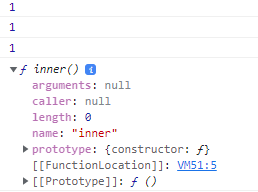GeeksforGeeks App
Open AppBrowser
Continue

# Closure in JavaScript

A closure is a feature of JavaScript that allows inner functions to access the outer scope of a function. Closure helps in binding a function to its outer boundary and is created automatically whenever a function is created. A block is also treated as a scope since ES6. Since JavaScript is event-driven so closures are useful as it helps to maintain the state between events.

Prerequisite:: Variable Scope in JavaScript

Lexical Scoping: A function scope’s ability to access variables from the parent scope is known as lexical scope. We refer to the parent function’s lexical binding of the child function as “lexically binding.”

Let’s see and understand closure through an example.

Example 1: This example shows the basic use of closure.

## Javascript

 `function` `foo() {``    ``let b = 1;``    ``function` `inner() {``        ``return` `b;``    ``}``    ``return` `inner;``}``let get_func_inner = foo();` `console.log(get_func_inner());``console.log(get_func_inner());``console.log(get_func_inner());`

Output: We can access the variable b which is defined in the function foo() through function inner() as the later preserves the scope chain of the enclosing function at the time of execution of the enclosing function i.e. the inner function knows the value of b through its scope chain.
This is closure in action that is inner function can have access to the outer function variables as well as all the global variables.Closure in JavaScript

Definition of Closure:

In programming languages, closures (also lexical closures or function closures) are techniques for implementing lexically scoped name binding in languages with first-class functions. Operationally, a closure is a record storing a function[a] together with an environment: a mapping associating each free variable of the function (variables that are used locally, but defined in an enclosing scope) with the value or reference to which the name was bound when the closure was created.[b]
-Wikipedia

or

In other words, closure is created when a child function keep the environment of the parent scope even after the parent function has already executed

Note: Closure is the concept of function + lexical environment in which function it was created. so every function declared within another function then it has access to the scope chain of the outer function and the variables created within the scope of the outer function will not get destroyed.

Now let’s look at another example.

Example 2: This example shows the basic use of closure.

## Javascript

 `function` `foo(outer_arg) {` `    ``function` `inner(inner_arg) {``        ``return` `outer_arg + inner_arg;``    ``}``    ``return` `inner;``}``let get_func_inner = foo(5);` `console.log(get_func_inner(4));``console.log(get_func_inner(3));`

Output: In the above example we used a parameter function rather than a default one. Not even when we are done with the execution of foo(5) we can access the outer_arg variable from the inner function. And on the execution of the inner function produce the summation of outer_arg and inner_arg as desired.

```9
8```

Now let’s see an example of closure within a loop.
In this example, we would store an anonymous function at every index of an array.

Example 3: This example shows the basic use of closure.

## Javascript

 `// Outer function``function` `outer() {``    ``let arr = [];``    ``let i;``    ``for` `(i = 0; i < 4; i++) {``        ``// storing anonymous function``        ``arr[i] = ``function` `() { ``return` `i; }``    ``}` `    ``// returning the array.``    ``return` `arr;``}` `let get_arr = outer();` `console.log(get_arr());``console.log(get_arr());``console.log(get_arr());``console.log(get_arr());`

Output: Did you guess the right answer? In the above code, we have created four closures that point to the variable i which is the local variable to the function outer. Closure doesn’t remember the value of the variable it only points to the variable or stores the reference of the variable and hence, returns the current value. In the above code when we try to update the value it gets reflected all because the closure stores the reference.

```4
4
4
4```

Let’s see the correct way to write the above code so as to get different values of i at different indexes.

Example 4: This example shows the basic use of closure

## Javascript

 `// Outer function``function` `outer() {``    ``function` `create_Closure(val) {``        ``return` `function` `() {``            ``return` `val;``        ``}``    ``}``    ``let arr = [];``    ``let i;``    ``for` `(i = 0; i < 4; i++) {``        ``arr[i] = create_Closure(i);``    ``}``    ``return` `arr;``}``let get_arr = outer();``console.log(get_arr());``console.log(get_arr());``console.log(get_arr());``console.log(get_arr());`

Output: In the above code we are updating the argument of the function create_Closure with every call. Hence, we get different values of i at different indexes.

```0
1
2
3```

Note: It may be slightly difficult to get the concept of closure at once but try experimenting with closure in different scenarios like for creating getter/setter, callbacks, and so on.

My Personal Notes arrow_drop_up
Related Tutorials